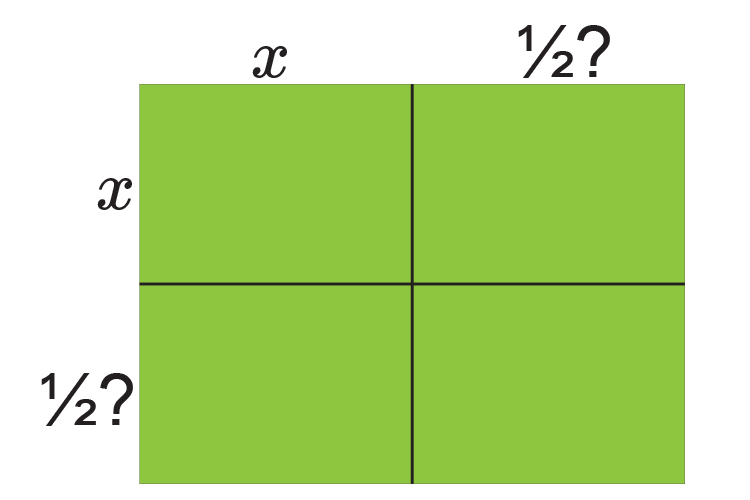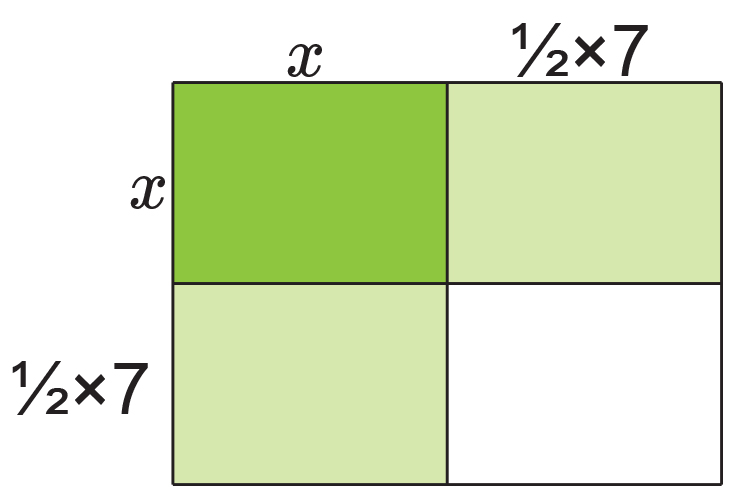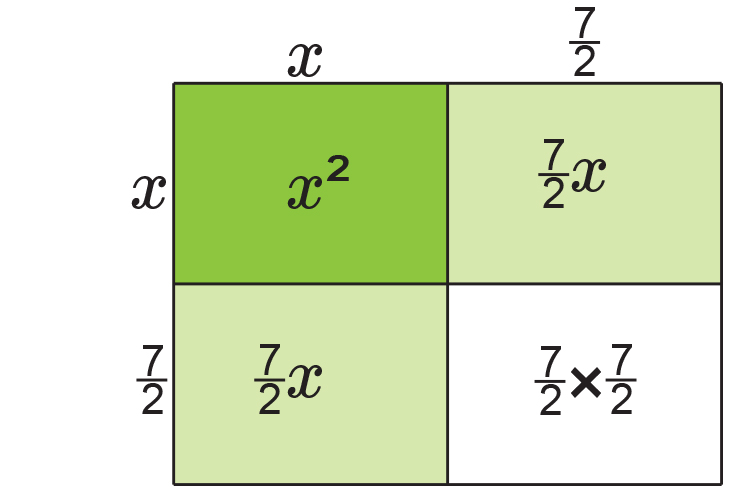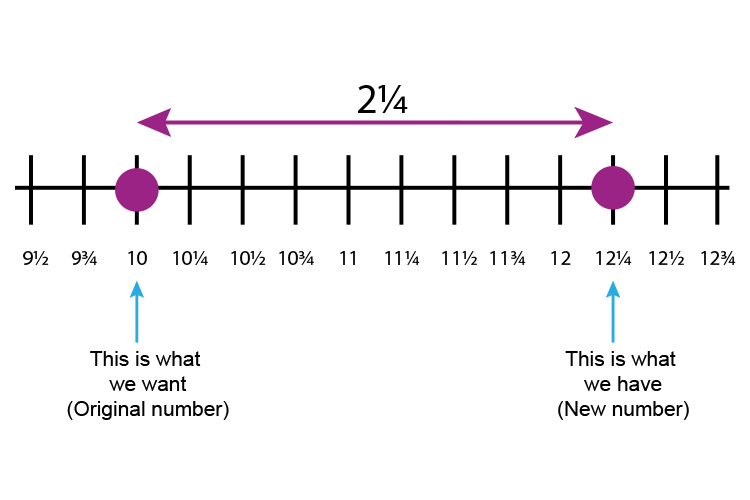# Completing the square - example 4

Complete the square x^2+7x+10=0

RememberFill inNOTE:

This is the same as (x+7/2)^2

If you add up each area you get:

x^2+7/2x+7/2x+7/2times7/2

x^2+7x+49/4

x^2+7x+12\1/4

Always plot this on a number lineOriginal number -  New number

10-12\1/4=-2\1/4

So     x^2+7x+10=0

Is the same as

(x+7/2)^2-2\1/4=0

(x+7/2)^2=2\1/4

x+7/2=+-sqrt(2\1/4)

x=-7/2+-sqrt(2\1/4)

Using a calculator

x=-3.5+-1.5

x=-3.5+1.5   and   -3.5-1.5

x=-2   and   -5

### Now check

x^2+7x+10=0

If x=-2              (-2)^2+7times(-2)+10=0

+4-14+10=0   Which is correct

If x=-5              (-5)^2+7times(-5)+10=0

25-35+10=0   Which is correct

The roots of x^2+7x+10=0   are x=-2   and  x=-5
This example has also been used in factorising quadratics (easy) and quadratic formula examples to show that the roots-2  and -5  can be found using any of these methods.